﻿ 神经网络在电动汽车电机寿命预测中的应用 Application of Neural Network in Reliability Prediction of Electric Vehicle Motor

Statistics and Application
Vol.06 No.05(2017), Article ID:22691,7 pages
10.12677/SA.2017.65056

Application of Neural Network in Reliability Prediction of Electric Vehicle Motor

Caijuan Wang

Changchun University of Technology, Changchun Jilin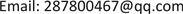Received: Oct. 28th, 2017; accepted: Nov. 10th, 2017; published: Nov. 16th, 2017ABSTRACT

The service reliability of the electric vehicle motor has been a consumer’s concerned topic. The service reliability of the electric vehicle motor data analysis are used the combination of ordinary least square and mathematical expectation empirical distribution function fitting method to estimate the parameters. At the same time, the neural network model and the mathematical expectation empirical distribution function are used to fit the estimation parameters, and the two methods are compared. The parameter estimation method usually used is affected by subjective factors. The benchmark of the reliability model is the empirical distribution function, and its precision affects the precision of the reliability model. In this paper, 18 electric vehicle motors with the same configuration are selected, and the failure data are collected in the same environment. The results show that the method of estimating parameters by neural network and empirical distribution function is more accurate and practical.

Keywords:Reliability, Empirical Function, Neural Network, Ordinary Least Square1. 引言

2. 研究方法

2.1. 最小二乘法

$Q=\sum {e}_{t}^{2}=\sum {\left({Y}_{t}-{\stackrel{^}{Y}}_{t}\right)}^{2}={\sum \left({Y}_{t}-{\stackrel{^}{\beta }}_{1}-{\stackrel{^}{\beta }}_{2}{X}_{t}\right)}^{2}$ (1)

$\left\{\begin{array}{c}\frac{\partial Q}{\partial {\stackrel{^}{\beta }}_{1}}=-2\sum \left({Y}_{t}-{\stackrel{^}{\beta }}_{1}-{\stackrel{^}{\beta }}_{2}{X}_{t}\right)=0\\ \frac{\partial Q}{\partial {\stackrel{^}{\beta }}_{2}}=-2\sum {X}_{t}\left({Y}_{t}-{\stackrel{^}{\beta }}_{1}-{\stackrel{^}{\beta }}_{2}{X}_{t}\right)=0\end{array}$ (2)

$\left\{\begin{array}{c}{\stackrel{^}{\beta }}_{2}=\frac{n\sum {X}_{t}{Y}_{t}-\sum {X}_{t}\sum {Y}_{t}}{n\sum {X}_{t}^{2}-{\left(\sum {X}_{t}\right)}^{2}}\\ {\stackrel{^}{\beta }}_{1}=\stackrel{¯}{Y}-{\stackrel{^}{\beta }}_{2}\stackrel{¯}{X}\end{array}$ (3)

${\stackrel{^}{Y}}_{t}={\stackrel{^}{\beta }}_{1}+{\stackrel{^}{\beta }}_{2}{X}_{t}$ (4)

2.2. 神经网络算法

3. 数据来源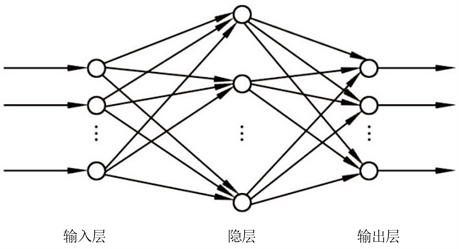Figure 1. The schematic diagram of neural networkTable 1. The motor failure time data of electric vehiclesFigure 2. Histogram and kernel density function diagram of motor vehicle fault data

4. 实证分析

$R\left({t}_{i}\right)=1-\frac{i}{N+1}$ (5)

4.1. 最小二乘法在电动汽车电机可靠性的预测中的应用

MATLAB是一个高级的处理、分析数值的软件，本文中利用MATLAB软件来实现曲线拟合问题的求解。采用MATLAB中提供的polyfit函数实现最小二乘拟合。其调用格式为：

[m q mu]=polyfit(x,y,n)

polyval(m,x)Table 2. Experience reliability function of electric vehicle motor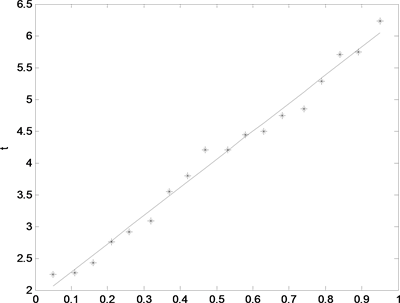Figure 3. Least square fitting graph

4.2. 神经网络在电动汽车电机可靠性的预测中的应用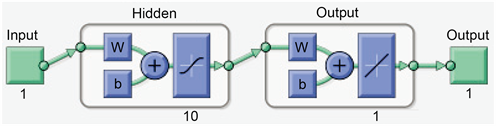Figure 4. Neural network input and output diagram of electric vehicle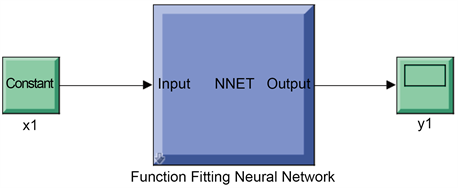Figure 5. Automatic generation of network Simulink model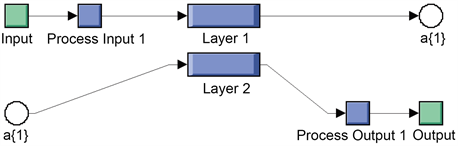Figure 6. Internal structure of neural network module by function fitting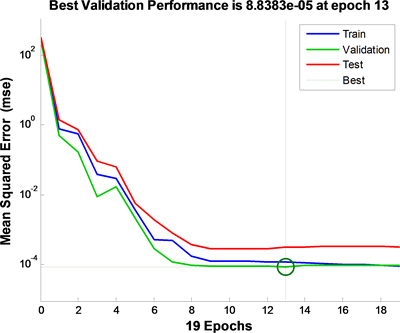Figure 7. The relation between the gradient and the mean variance of the training data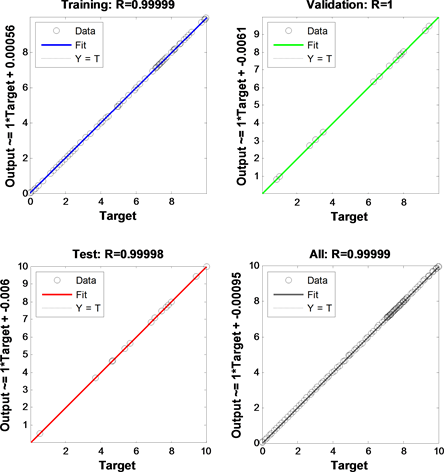Figure 8. Regression diagram

4.3. 两种拟合方法的比较

5. 结论与建议

Application of Neural Network in Reliability Prediction of Electric Vehicle Motor[J]. 统计学与应用, 2017, 06(05): 501-507. http://dx.doi.org/10.12677/SA.2017.65056

1. 1. 李峰, 谢俊, 兰金波, 等. 智能变电站继电保护配置的展望与探讨[J]. 电力自动化设备, 2012, 32(2): 122-126.

2. 2. 张春合, 陆征军, 李九虎, 等. 数字化变电站的保护配置方案和应用[J]. 电力自动化设备, 2011, 31(6): 122-125.

3. 3. 郭勇基. 可靠性工程原理[M]. 北京: 清华大学出版社, 2002.

4. 4. Gomes, H.M. and Awruch, A.M. (2004) Comparison of Response Surface and Neural Network with Other Methods for Structural Reliability Analysis. Structural Safety, 26, 49-67.

5. 5. Kaymaz, I. (2005) Application of Kriging Method to Structural Reliability Problems. Structural Safety, 27, 25-48. https://doi.org/10.1016/j.strusafe.2004.09.001